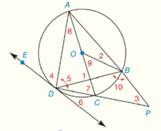Chapter 6.CR, Problem 30CR### Elementary Geometry for College St...

6th Edition
Daniel C. Alexander + 1 other
ISBN: 9781285195698

#### Solutions

Chapter
Section### Elementary Geometry for College St...

6th Edition
Daniel C. Alexander + 1 other
ISBN: 9781285195698
Textbook Problem
1 views

# G i v e n :                       ⊙ O   w i t h   d i a m e t e r   A C -   a n d   t a n g e n t   D E ⃡                                                                       m A D ^ = 136 °   a n d   m B C ^ = 50 °                 F i n d :                           T h e   m e a s u r e s   o f   ∠ 1   t h r o u g h   ∠ 10To determine

To Find: The measures of 1 through 10, if O with diameter AC- and tangent DE. mAD^=136° and mBC^=50°.Explanation

Concept:

Definition:

A central angle of a circle is an angle whose vertex is the center of the circle and whose sides are radii of the circle.

Definition:

An inscribed angle of a circle is an angle whose vertex is a point on the circle and whose sides are chords of the circle.

Theorem 6.1.2:

The measure of an inscribed angle of a circle is one-half the measure of its intercepted arc.

Theorem 6.1.8:

Congruent chords are located at the same distance from the center of a circle.

Theorem 6.1.9:

Angle inscribed in a semicircle is a right angle.

Theorem 6.2.2:

The measures of an angle formed by two chords that intersect within a circle is one-half the sum of the measures of the arcs intercepted by the angle and its vertical angle.

Theorem 6.2.4:

The measure of an angle formed by a tangent and a chord drawn to the point of tangency is one-half the measure of the intercepted arc.

Theorem 6.2.5:

The measure of an angle formed when two secants at a point outside the circle is one-half the difference of the measures of the two intercepted arcs.

Calculation:

Given O with diameter AC- and tangent DE. mAD^=136° and mBC^=50°.

Since mBC^=50°, its central angle is 9=50°.

By Theorem 6.1.9:

Angle inscribed in a semicircle is a right angle.

So, 5=90°

By Theorem 6.2.4:

The measure of an angle formed by a tangent and a chord drawn to the point of tangency is one-half the measure of the intercepted arc.

So, 6=12mDC^ and 4=12AOD=12×136°=68°

Also, 7=12AOD=12×136°=68°

Since sum of the three angles in ADC=180°

5+7+8=180°.

90+68+8=180°.

158°+8=180°.

8=180°-158°

8=22°

And 6=12mDC^=442=22°

By Theorem 6.2.2:

The measures of an angle formed by two chords that intersect within a circle is one-half the sum of the measures of the arcs intercepted by the angle and its vertical angle

### Still sussing out bartleby?

Check out a sample textbook solution.

See a sample solution

#### The Solution to Your Study Problems

Bartleby provides explanations to thousands of textbook problems written by our experts, many with advanced degrees!

Get Started

#### Convert the expressions in Exercises 6584 to power form. x23

Finite Mathematics and Applied Calculus (MindTap Course List)

#### Simplify each of the following so that only positive exponents remain.

Mathematical Applications for the Management, Life, and Social Sciences

#### In Exercises 63-68, rationalize the denominator. 66. 5x23x

Applied Calculus for the Managerial, Life, and Social Sciences: A Brief Approach

#### The graph at the right has a local maximum at x ______. a) 1, 3, and 5 b) 2 c) 4 d) 2 and 4

Study Guide for Stewart's Single Variable Calculus: Early Transcendentals, 8th

#### True or False: is monotonic.

Study Guide for Stewart's Multivariable Calculus, 8th